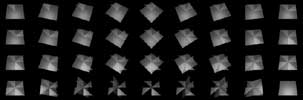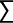cubeLife.org

# Unique matrix positions in magic square pattern rotation and reflection

The following explains the issue inherent in deciding whether rotations and reflections should be considered as original magic squares on a fixed matrix, or as a smaller separate number of fixed pattern groups based solely on numerical relation and translated through rotation and reflection. The case is argued for the latter for reasons outlined in the summary below, and a proposal is made for a further simplification based on the unique positions remaining after rotation and reflection of a mogic square.

## General notes:

This description is written by a digital artist with an interest in magic square and cube patterns, not a mathematician. Thanks to Simon Nee at Loughborough University Computer Human Interface Department for his input on refining the formula, and for other work. Thanks also to Alan Grogono, Dave Harper and Charles Kelley (and their magic square websites) who have responded with helpful and informed comments.

## Summary

This inquiry begins with the assertion that - for any given single magic square of any order based only on rotation and reflection - the number of permutations is, of course, 8 - the Dn or dihedral group of symmetries for a square (where n is the order of the regular-sided polygon rotated). However, this raises a further, more fundamental issue of the number of unique positions within a magic square matrix that any number can occupy, once those eliminated by the symmetry group are excluded.

If magic squares are regarded as sets of integers in a fixed matrix in which each position is unique and static, the eight rotations and reflections of any magic square pattern within that matrix must be regarded as separate entities. However, the generally held position for magic squares disreguards these rotations and reflections, as do formulae for determining the rules for various magic square permutations. But an idealised individual square is treated as a single group where the eight rotations and reflections form a (trivial in the existing view) group of symmetries, there are still implications for such rules, outlined below.

## Argument

If any number in a magic square is rotated and reflected through the square's group of eight symmetries, it can be seen to occupy either 1, 4 or 8 unique positions, depending on its initial location in the `n x n` matrix. In other words, once rotations and reflections are allowed for in any magic square pattern, the possible locations of any single number in the matrix covers only a limited area. For example, when attempting to determine the location of any number `n..n^2` in any `n x n` magic square, a number falling outside this area can be seen to be a result of reflection and rotation acting upon the matrix.

The simple formula given calculates (for any one magic square pattern) the number of unique positions that any number in the sequence `n..n^2` may occupy in a fixed matrix without its position being the result of either reflection, rotation or both. My inquiry is to find out whether this very basic premise has been explored in cases where the total number of possible magic squares for a certain order is given (at the time of writing this was known only up to order 5), and whether it may be of use in determining the same for higher orders. So although the following is a conclusion drawn only from those rules governing rotation and reflection, it may have implications for the process of discovering all possible integer combinations for a given order of square, particularly if combined with other, already known, methods of simplification (see 'conclusion', below).

## 1. The effect of group rotations and reflections on magic square symmetry

An order 3 (or Lo Shu) square is recognised as having only one pattern. If that pattern is rotated or reflected as a group, the possible permutations on a fixed number grid total eight (including the original). So for instance, position `*` in the following pattern:

The position at `*` by rotation can also occupy three further positions:

``````
* B C     G D *      I H G      C F I
D E F ->  H E B  or  F E D  or  B E H
G H I     I F C      C B *      * D G
``````

each of which by reflection also occupy:

``````
C B *     * D G      G H I      I F C
F E D     B E H      D E F      H E B
I H G     C F I      * B C      G D *
``````

the integers represented by the letters A-I above retain their pattern of relation to each other throughout, so the magic property of the integer matrix is maintained. The consensus correctly maintains that there is only one order 3 magic square. If this is so, then all the reflections and rotations (7 for each individual n x n pattern) become trivial subsets or symmetries of the original pattern. So far, this is already established thinking.

Algorithms (for instance) for determining the total possible number of magic squares for any given order need then, as a matter of course, to account for the seven possible rotational and reflectional variations of each original pattern. It follows that any given total needs to state whether it includes the seven variations or symmetries of each magic square pattern or group of patterns. This is also the assumed rule.

It follows that if any magic square of order `n` is regarded as an arrangement of `n^2` integers in a fixed matrix `n x n` (so that any row, column or diagonal of `n` integers yields the same number), then all possible variations are valid, since the matrix itself cannot be reflected or rotated, therefore each arrangement is unique. But if magic squares are regarded as an arrangement of integers in a matrix according to a particular sequence or pattern of relationship with each other, then the relational pattern itself becomes the valid mode of classification, superceding the fixed matrix on which that pattern appears. The pattern must thus remain intact throughout rotation and reflection i.e. every integer retains its position relative to the others in the group pattern so that, regardless of how rotation and reflection change its own position in the matrix, the 'magic' properties of the square are preserved. This occurs because each transformation is applied simultaneously to the position of every integer.

## 2. Unique positions in an `n x n` matrix

If the 7 extra possible rotations and reflections for any one pattern are discounted, the remaining positions any integer can occupy within the `n x n` matrix of that pattern take up a rough one-eighth slice of the square. For odd squares this includes positions along the axes of translation. The exact number of original positions for any one number in a magic square can be therefore expressed by the formula:

``````
( (n^2+4n+3)/8
(  n is odd
N = (
( (n^2+2n)/8
(  n is even
``````

where `N` is the number of unique positions and `n` is the order of the square.

Which simplifies as:`` n/2 (n is even) n+1/2 (n is odd)``

for example

``````
n=4

(16+8)/8 = 3
``````

so an even square of order 4 has three possible unique matrix positions that any number (represented below by the asterisk `*`) may occupy:

``````
* * 0 0
0 * 0 0
0 0 0 0
0 0 0 0
``````

and an order 5 has six possible unique matrix positions:

``````
n=5

(25+20+3)/8 = 6
* * * 0 0
0 * * 0 0
0 0 * 0 0
0 0 0 0 0
0 0 0 0 0
``````

These unique positions arise from the symmetry group for a square acting upon the set of a magic square number matrix.

## Conclusion: the search for an effective magic square classification

Formulating a proof for the above is naturally the next task… until then, however…

It is presumed that the general consensus is that reflections and rotations do not constitute separate magic squares, with the rule observed in the case of the therefore single order 3 magic square being applied to higher orders. This results in the accepted totals known to date up to and including order 5.

The following observations follow on from the exclusion of reflections and rotations by exploring further methods for grouping magic square permutations of any order. Logical groupings greatly simplify the process of understanding relationships between the immense number of variations involved in higher-order magic squares - for an example, see this page on the simplification of 4x4 patterns by Alan Grogono.

Of the number of ways in which we can view and classify magic square patterns, the simplest graphical method is to connect the integers in sequence from `1..n2` with a line passing from `1, 2, 3, ... n^2` to produce what is known as the 'magic line'. Using this visual method it can soon be seen, for instance, that for order 4 squares there exists a finite number of pattern groups, which are in themselves either bilaterally symmetrical along one axis, or asymmetrical. Further, these may be seen to fall into parent and child patterns according to other known rules, such as forming one magic square from another by transposing rows and/or columns; or by repositioning the numbers in the matrix according to numerical rules that depend upon the square's order. 'Complete' or 'pan-magic' squares can also be made into an extended magic carpet that produces related groups. In either case it can be shown that pattern families tend to overlap. The combination of the latter with the 'unique position' formula above may provide greater degrees of simplification in determining such family groups.

Dave Everitt, Feb 29 2000 (revised March 15, May 15, 2000; March 23 2002, October 16 2009, April 7 2011)